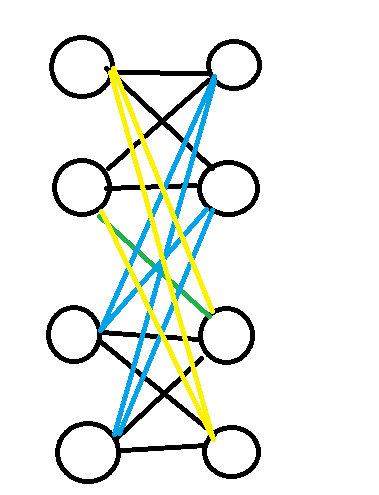WA 了一次，因为没有判当 $A_i + 10A_{i-1} > 20$ 的时候，而 $S$ 只预处理到了 $20$ 。

## B Geometers Anonymous Club

WA 了一次，因为数组没开够 $3 \times 10^5$ 。

## H Extending Set of Points## J Fibonotci

• 修改一个位置会对应修改的是 $s_i,s_{(i+1)\bmod k}$ 。也就是说如果修改序列的末尾，会导致下一个块也被改了，这种情况需要在每个块特判开头的上一个位置是否被修改。同样需要特判修改最后一个位置的时候不会继续修改开头。
• 如果 $k$ 所在的就是开头块，那么要特判掉 $0$ 的位置。同样对于任何开头块都要从 $1$ 开始乘。
• 特判 $k=0,k=1,P=1$ 的情况。
• 记得各种地方开 long long

## L Two Permutations

a这个问题可以用 Hash 来解决。具体来说，我们可以设 Hash 值是 $\sum a_i B^i$ 。那么在 $b$ 序列中，区间的 Hash 值即是 $\sum i B^{rnk_i}$ 这个东西。如果这两个相同，说明这个区间最小的在 $a_1$ 位，次小在 $a_2$ 位 $\dots$ ，也就是满足了上面说的那个条件。